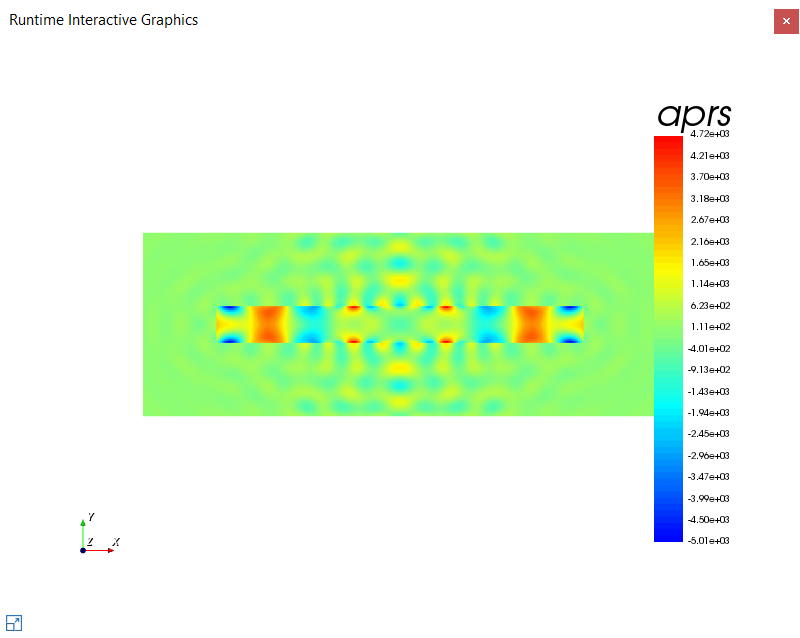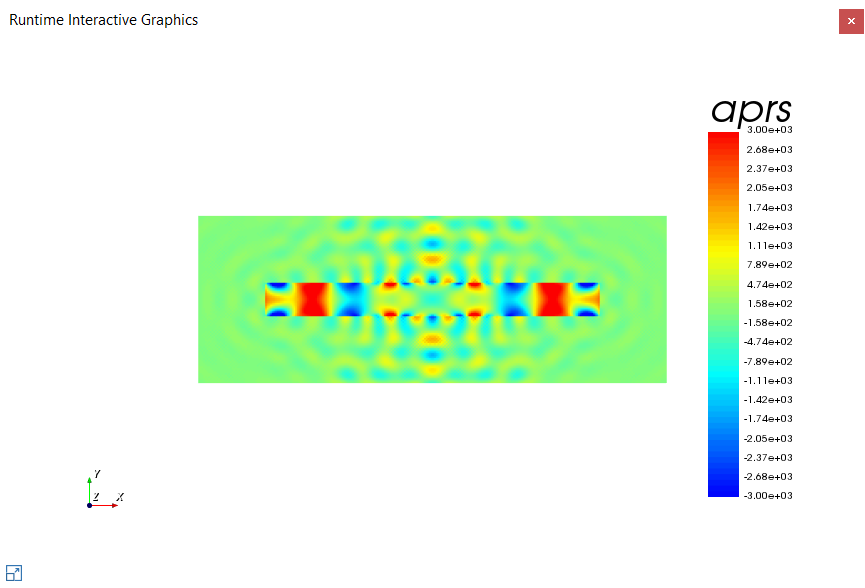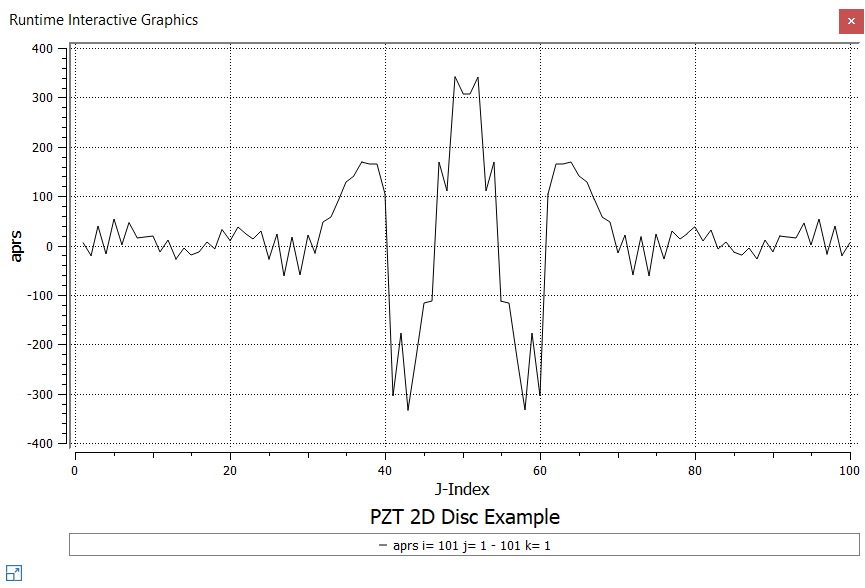# Plotting data fields

Data fields in OnScale refer to snapshots of particular qualities at specific times in the model. The CALC command is used in OnScale as a means to specify secondary field variables and energy quantities to be computed and stored during execution. Secondary field variables are nodal and elemental quantities that the computational algorithm does not require to be stored during the computation. Secondary field variable require very little CPU time, but require large amounts of memory to store requested data.

A few examples will be presented showing some of the ways time histories can be plotted during execution, all examples were done by modifying the PZT Disk 2D example in our Examples section:

``` calc
pres acoustic 		/* Calculate acoustic pressure
disp   			/* Calculate X and Y displacements
max aprs none pmax      /* Calculate maximum pressure field
max ydsp none ydmax     /* Calculate maximum pressure field
end
```

displacement, pressure and acoustic pressure data has been requested via the CALC command.

These examples will show how to plot the requested data fields. These requested data fields can also be plotted wit time histories however for these particular example they will not be plotted, more information on plotting time histories can be found here. Some initial set up is required before using the GRPH command to plot the requested data.

```prcssymb #get { step } timestep        /* Extract calculated timesteps from PRCS command    symb simtime = 40 / \$freqint       /* Simulation time - 40 cycles / @ 1 MHzsymb nexec = \$simtime / \$step      /* Determine how many timesteps to run full modelsymb nloops = 40                   /* Plotting snapsots during model executionsymb nexec2 = \$nexec / \$nloopsgrph     arrow off                     /* Turn off arrows      line off                      /* Turn off mesh lines     set imag mpeg                 /* Set up image capture for movie file generation     nvew 1                        /* Set up 1 plotting widows      endproc plot saveexec \$nexec2 ** INSERT GRPH COMMAND HERE **
end\$ proc		/* End of Procedure Code
c Run model by calling 'plot' procedure 'nloops' times
proc plot \$nloops

term	/* Pause model - Allow user to call procedure 'plot' from console window to run model for longer```

The acoustic pressure in a model can be plotted using the following:

```grph
plot aprs	        /* Plot calculated data array
imag		/* Snapshot of Graphics window for Movie generation
end```The scales of the plots are, by default, scaled from max to min but they can be modified using the “rang” option:

`grph     plot aprs rang -3.e3 3.e3       /* plot pressure with a scale limitation     end`

Notice how the scale of the plot has changed to the modified scaleSlices of fields may be plotted using the “plot” sub command in the following manner:

```grph
plot aprs i \$i2        /* plot a slice of pressure along \$i2     end```The model used as an example is 2D but multiple slices or cross sections of a 3D model can be plotted using the PLOT subcommand in the following manner:

```grph
plot pres i 2 pres j 5 pres k 1```

This will give 2D slices of pressure, all on the same plot, at i = 2, then j = 5 then k = 1.

These are very basic examples of how to use your data. For more help on the GRPH command or for more advanced subcommands that accompany GRPH please refer to OnScale help documentation that can be accessed via the help tab in the application's ribbon.